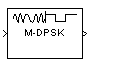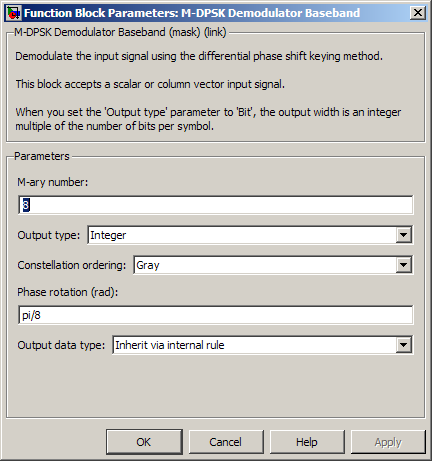Documentation

M-DPSK Demodulator Baseband

Demodulate DPSK-modulated data

Library

PM, in Digital Baseband sublibrary of ModulationDescription

The M-DPSK Demodulator Baseband block demodulates a signal that was modulated using the M-ary differential phase shift keying method. The input is a baseband representation of the modulated signal. The input and output for this block are discrete-time signals. This block accepts a scalar-valued or column vector input signal. For information about the data types each block port supports, see the Supported Data Types table on this page.

The M-ary number parameter, M, is the number of possible output symbols that can immediately follow a given output symbol. The block compares the current symbol to the previous symbol. The block's first output is the initial condition of zero (or a group of zeros, if the Output type parameter is set to Bit) because there is no previous symbol.

Integer-Valued Signals and Binary-Valued Signals

If you set the Output type parameter to Integer, then the block demodulates a phase difference of

θ + 2πk/M

to k, where θ represents the Phase rotation parameter and k represents an integer between 0 and M-1.

When you set the Output type parameter to Bit, the block outputs binary-valued signals that represent integers. The block represents each integer using a group of K = log2(M) bits, where K represents the number of bits per symbol. The output vector length must be an integer multiple of K.

In binary output mode, the symbols can be either binary-demapped or Gray-demapped. The Constellation ordering parameter indicates how the block maps an integer to a corresponding group of K output bits. See the reference pages for the M-DPSK Modulator Baseband and M-PSK Modulator Baseband blocks for details.

Dialog BoxM-ary number

The number of possible modulated symbols that can immediately follow a given symbol.

Output type

Determines whether the output consists of integers or groups of bits.

Constellation ordering

Determines how the block maps each integer to a group of output bits.

This phase difference between the current and previous modulated symbols that results in an output of zero.

Output data type

When the parameter is set to 'Inherit via internal rule' (default setting), the block will inherit the output data type from the input port. The output data type will be the same as the input data type if the input is of type single or double.

For integer outputs, this block can output the data types int8, uint8, int16, uint16, int32, uint32, single, and double. For bit outputs, output can be int8, uint8, int16, uint16, int32, uint32, boolean, single, or double.

Supported Data Types

PortSupported Data Types

Input

• Double-precision floating point

• Single-precision floating point

Output

• Double-precision floating point

• Single-precision floating point

• Boolean when Output type set to Bit

• 8-, 16-, and 32-bit signed integers

• 8-, 16-, and 32-bit unsigned integers

Pair Block

M-DPSK Modulator Baseband

References

 Pawula, R. F., "On M-ary DPSK Transmission Over Terrestrial and Satellite Channels," IEEE Transactions on Communications, Vol. COM-32, July 1984, 752-761.